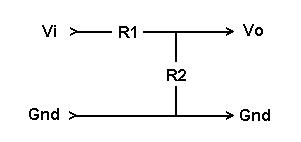Daqarta
Data AcQuisition And Real-Time Analysis
Scope - Spectrum - Spectrogram - Signal Generator
Software for WindowsThe following is from the Daqarta Help system:

# Voltage Dividers

A simple 2-resistor voltage divider can function as a fixed attenuator to reduce a signal level that is too large.Given the input voltage Vi and the two resistors R1 and R2, the output voltage Vo is:

Vo = Vi * R2 / (R1 + R2)

The fractional voltage reduction K is:

K = Vo / Vi = R2 / (R1 + R2)

To get a specified output voltage from a known input voltage, pick one of the resistor values and solve for the other:

R2 = Vo * R1 / (Vi - Vo)

R1 = R2 * (Vi - Vo) / Vo

Or, if you know the desired fraction K and want to find the resistors:

R2 = K * R1 / (1 - K)

R1 = R2 * (1 - K) / K

The ratio of the two resistors needed for a given reduction is:

R2/R1 = K / (1 - K)

R2/R1 = Vo / (Vi - Vo)

To determine the input voltage that produces a given output voltage from a known divider:

Vi = Vo * (R1 + R2) / R2

The reduction ratio K is normally used for range changes, such as 0.10 for a 10-to-1 reduction. However, for audio work you may want to obtain a specific reduction in dB. (See dB From Voltages and Working With dB.)

The dB corresponding to a given voltage ratio is:

dB = 20 * log10(Vo / Vi)

dB = 20 * log10(K)

where log is the base-10 (common) logarithm. For voltage dividers, note that K is always less than 1, so the dB value will always be negative.

To find the ratio K corresponding to a given dB value, use the following formula. Remember to use a negative value for dB:

K = 10^(dB/20)

Typical dB values and corresponding voltage ratios:

```-1 = 0.891251
-2 = 0.794328
-3 = 0.707946
-6 = 0.501187
-10 = 0.316228
-20 = 0.100000
-30 = 0.031623
-40 = 0.010000
-60 = 0.001000
-80 = 0.000100
-100 = 0.000010
-120 = 0.000001
```GO: